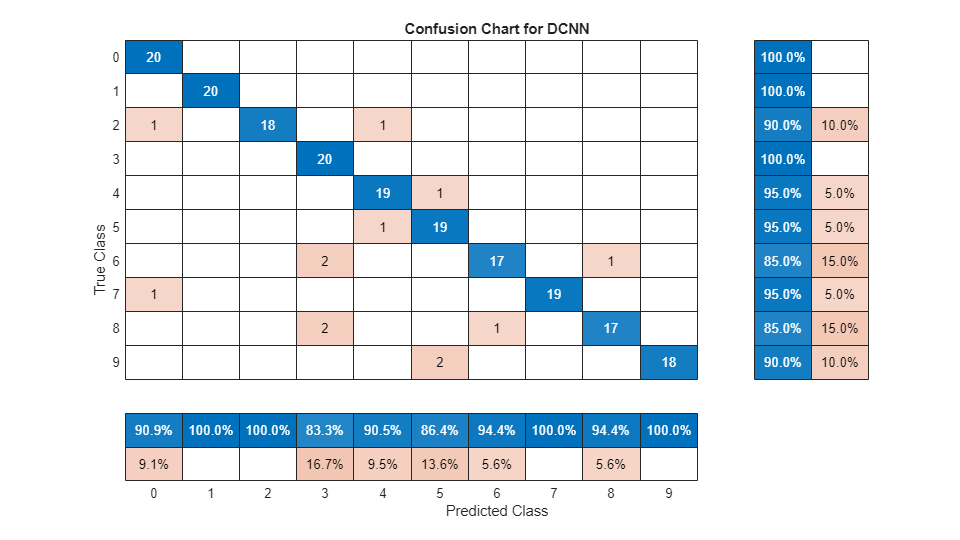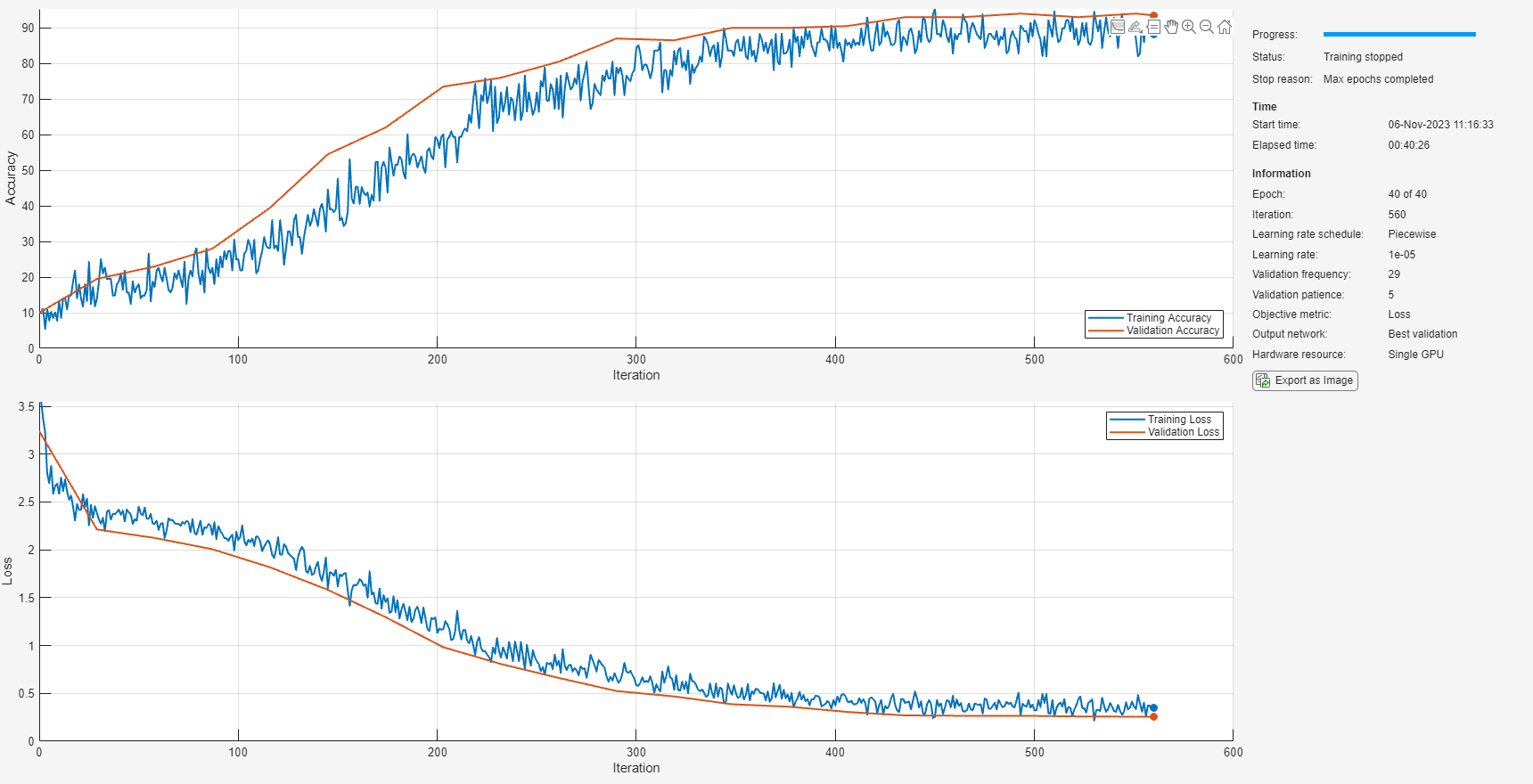# Train Spoken Digit Recognition Network Using Out-of-Memory Audio Data

This example trains a spoken digit recognition network on out-of-memory audio data using a transformed datastore. In this example, you apply a random pitch shift to audio data used to train a convolutional neural network (CNN). For each training iteration, the audio data is augmented using the audioDataAugmenter object and then features are extracted using the audioFeatureExtractor object. The workflow in this example applies to any random data augmentation used in a training loop. The workflow also applies when the underlying audio data set or training features do not fit in memory.

### Data

Download the Free Spoken Digit Data Set (FSDD). FSDD consists of 2000 recordings of four speakers saying the numbers 0 through 9 in English.

```url = 'https://ssd.mathworks.com/supportfiles/audio/FSDD.zip'; downloadFolder = tempdir; datasetFolder = fullfile(downloadFolder,'FSDD'); if ~exist(datasetFolder,'dir') disp('Downloading FSDD...') unzip(url,downloadFolder) end```

Create an `audioDatastore` that points to the dataset.

```pathToRecordingsFolder = fullfile(datasetFolder,'recordings'); location = pathToRecordingsFolder; ads = audioDatastore(location);```

The helper function, `helperGenerateLabels`, creates a categorical array of labels from the FSDD files. The source code for `helpergenLabels` is listed in the appendix. Display the classes and the number of examples in each class.

`ads.Labels = helpergenLabels(ads);`
```Starting parallel pool (parpool) using the 'local' profile ... Connected to the parallel pool (number of workers: 8). ```
`summary(ads.Labels)`
``` 0 200 1 200 2 200 3 200 4 200 5 200 6 200 7 200 8 200 9 200 ```

Split the FSDD into training and test sets. Allocate 80% of the data to the training set and retain 20% for the test set. You use the training set to train the model and the test set to validate the trained model.

```rng default ads = shuffle(ads); [adsTrain,adsTest] = splitEachLabel(ads,0.8); countEachLabel(adsTrain)```
```ans=10×2 table Label Count _____ _____ 0 160 1 160 2 160 3 160 4 160 5 160 6 160 7 160 8 160 9 160 ```
`countEachLabel(adsTest)`
```ans=10×2 table Label Count _____ _____ 0 40 1 40 2 40 3 40 4 40 5 40 6 40 7 40 8 40 9 40 ```

### Reduce Training Dataset

To train the network with the entire dataset and achieve the highest possible accuracy, set `reduceDataset` to false. To run this example quickly, set `reduceDataset` to true.

```reduceDataset ="false"; if reduceDataset == "true" adsTrain = splitEachLabel(adsTrain,2); adsTest = splitEachLabel(adsTest,2); end```

### Transformed Training Datastore

#### Data Augmentation

Augment the training data by applying pitch shifting with an `audioDataAugmenter` object.

Create an `audioDataAugmenter`.` `The augmenter applies pitch shifting on an input audio signal with a 0.5 probability. The augmenter selects a random pitch shifting value in the range [-12 12] semitones.

```augmenter = audioDataAugmenter('PitchShiftProbability',.5, ... 'SemitoneShiftRange',[-12 12], ... 'TimeShiftProbability',0, ... 'VolumeControlProbability',0, ... 'AddNoiseProbability',0, ... 'TimeShiftProbability',0);```

Set custom pitch-shifting parameters. Use identity phase locking and preserve formants using spectral envelope estimation with 30th order cepstral analysis.

`setAugmenterParams(augmenter,'shiftPitch','LockPhase',true,'PreserveFormants',true,'CepstralOrder',30);`

Create a transformed datastore that applies data augmentation to the training data.

```fs = 8000; adsAugTrain = transform(adsTrain,@(y)deal(augment(augmenter,y,fs).Audio{1}));```

#### Mel Spectrogram Feature Extraction

The CNN accepts mel-frequency spectrograms.

Define parameters used to extract mel-frequency spectrograms. Use 220 ms windows with 10 ms hops between windows. Use a 2048-point DFT and 40 frequency bands.

```frameDuration = 0.22; hopDuration = 0.01; params.segmentLength = 8192; segmentDuration = params.segmentLength*(1/fs); params.numHops = ceil((segmentDuration-frameDuration)/hopDuration); params.numBands = 40; frameLength = round(frameDuration*fs); hopLength = round(hopDuration*fs); fftLength = 2048;```

Create an `audioFeatureExtractor` object to compute mel-frequency spectrograms from input audio signals.

```afe = audioFeatureExtractor('melSpectrum',true,'SampleRate',fs); afe.Window = hamming(frameLength,'periodic'); afe.OverlapLength = frameLength-hopLength; afe.FFTLength = fftLength;```

Set the parameters for the mel-frequency spectrogram.

`setExtractorParams(afe,'melSpectrum','NumBands',params.numBands,'FrequencyRange',[50 fs/2],'WindowNormalization',true);`

Create a transformed datastore that computes mel-frequency spectrograms from pitch-shifted audio data. The helper function, `getSpeechSpectrogram` (see appendix), standardizes the recording length and normalizes the amplitude of the audio input. `getSpeechSpectrogram` uses the `audioFeatureExtractor` object (afe) to obtain the log-based mel-frequency spectrograms.

`adsSpecTrain = transform(adsAugTrain, @(x)getSpeechSpectrogram(x,afe,params));`

#### Training Labels

Use an `arrayDatastore` to hold the training labels.

`labelsTrain = arrayDatastore(adsTrain.Labels);`

#### Combined Training Datastore

Create a combined datastore that points to the mel-frequency spectrogram data and the corresponding labels.

`tdsTrain = combine(adsSpecTrain,labelsTrain);`

### Validation Data

The validation dataset fits into memory and you precompute validation features using the helper function `getValidationSpeechSpectrograms` (see appendix).

`XTest = getValidationSpeechSpectrograms(adsTest,afe,params);`

Get the validation labels.

`YTest = adsTest.Labels;`

### Define CNN Architecture

Construct a small CNN as an array of layers. Use convolutional and batch normalization layers, and downsample the feature maps using max pooling layers. To reduce the possibility of the network memorizing specific features of the training data, add a small amount of dropout to the input to the last fully connected layer.

```sz = size(XTest); specSize = sz(1:2); imageSize = [specSize 1]; numClasses = numel(categories(YTest)); dropoutProb = 0.2; numF = 12; layers = [ imageInputLayer(imageSize,'Normalization','none') convolution2dLayer(5,numF,'Padding','same') batchNormalizationLayer reluLayer maxPooling2dLayer(3,'Stride',2,'Padding','same') convolution2dLayer(3,2*numF,'Padding','same') batchNormalizationLayer reluLayer maxPooling2dLayer(3,'Stride',2,'Padding','same') convolution2dLayer(3,4*numF,'Padding','same') batchNormalizationLayer reluLayer maxPooling2dLayer(3,'Stride',2,'Padding','same') convolution2dLayer(3,4*numF,'Padding','same') batchNormalizationLayer reluLayer convolution2dLayer(3,4*numF,'Padding','same') batchNormalizationLayer reluLayer maxPooling2dLayer(2) dropoutLayer(dropoutProb) fullyConnectedLayer(numClasses) softmaxLayer classificationLayer('Classes',categories(YTest)); ];```

Set the hyperparameters to use in training the network. Use a mini-batch size of 50 and a learning rate of 1e-4. Specify 'adam' optimization. To use the parallel pool to read the transformed datastore, set `DispatchInBackground` to `true`. For more information, see `trainingOptions` (Deep Learning Toolbox).

```miniBatchSize = 50; options = trainingOptions('adam', ... 'InitialLearnRate',1e-4, ... 'MaxEpochs',30, ... 'LearnRateSchedule',"piecewise",... 'LearnRateDropFactor',.1,... 'LearnRateDropPeriod',15,... 'MiniBatchSize',miniBatchSize, ... 'Shuffle','every-epoch', ... 'Plots','training-progress', ... 'Verbose',false, ... 'ValidationData',{XTest, YTest},... 'ValidationFrequency',ceil(numel(adsTrain.Files)/miniBatchSize),... 'ExecutionEnvironment','gpu',... 'DispatchInBackground', true);```

Train the network by passing the transformed training datastore to `trainNetwork`.

`trainedNet = trainNetwork(tdsTrain,layers,options);`

Use the trained network to predict the digit labels for the test set.

```[Ypredicted,probs] = classify(trainedNet,XTest); cnnAccuracy = sum(Ypredicted==YTest)/numel(YTest)*100```
```cnnAccuracy = 96.2500 ```

Summarize the performance of the trained network on the test set with a confusion chart. Display the precision and recall for each class by using column and row summaries. The table at the bottom of the confusion chart shows the precision values. The table to the right of the confusion chart shows the recall values.

```figure('Units','normalized','Position',[0.2 0.2 0.5 0.5]); ccDCNN = confusionchart(YTest,Ypredicted); ccDCNN.Title = 'Confusion Chart for DCNN'; ccDCNN.ColumnSummary = 'column-normalized'; ccDCNN.RowSummary = 'row-normalized';```### Appendix: Helper Functions

```function Labels = helpergenLabels(ads) % This function is only for use in this example. It may be changed or % removed in a future release. files = ads.Files; tmp = cell(numel(files),1); expression = "[0-9]+_"; parfor nf = 1:numel(ads.Files) idx = regexp(files{nf},expression); tmp{nf} = files{nf}(idx); end Labels = categorical(tmp); end %------------------------------------------------------------ function X = getValidationSpeechSpectrograms(ads,afe,params) % This function is only for use in this example. It may changed or be % removed in a future release. % % getValidationSpeechSpectrograms(ads,afe) computes speech spectrograms for % the files in the datastore ads using the audioFeatureExtractor afe. numFiles = length(ads.Files); X = zeros([params.numBands,params.numHops,1,numFiles],'single'); for i = 1:numFiles x = read(ads); spec = getSpeechSpectrogram(x,afe,params); X(:,:,1,i) = spec; end end %-------------------------------------------------------------------------- function X = getSpeechSpectrogram(x,afe,params) % This function is only for use in this example. It may changed or be % removed in a future release. % % getSpeechSpectrogram(x,afe) computes a speech spectrogram for the signal % x using the audioFeatureExtractor afe. X = zeros([params.numBands,params.numHops],'single'); x = normalizeAndResize(single(x),params); spec = extract(afe,x).'; % If the spectrogram is less wide than numHops, then put spectrogram in % the middle of X. w = size(spec,2); left = floor((params.numHops-w)/2)+1; ind = left:left+w-1; X(:,ind) = log10(spec + 1e-6); end %-------------------------------------------------------------------------- function x = normalizeAndResize(x,params) % This function is only for use in this example. It may change or be % removed in a future release. L = params.segmentLength; N = numel(x); if N > L x = x(1:L); elseif N < L pad = L-N; prepad = floor(pad/2); postpad = ceil(pad/2); x = [zeros(prepad,1) ; x ; zeros(postpad,1)]; end x = x./max(abs(x)); end ```

## SupportGet trial now